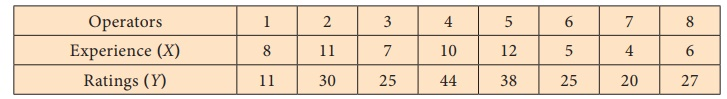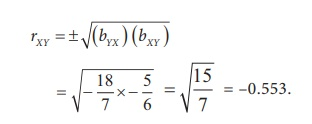Home | | Statistics 12th Std | Properties of Regression Coefficients

# Properties of Regression Coefficients

Correlation coefficient is the geometric mean between the regression coefficients.

PROPERTIES OF REGRESSION COEFFICIENTS

1. Correlation coefficient is the geometric mean between the regression coefficients.2. It is clear from the property 1, both regression coefficients must have the same sign. i.e., either they will positive or negative.

3. If one of the regression coefficients is greater than unity, the other must be less than unity.

4. The correlation coefficient will have the same sign as that of the regression coefficients.

5. Arithmetic mean of the regression coefficients is greater than the correlation coefficient.6. Regression coefficients are independent of the change of origin but not of scale.

## Properties of regression equation

1. If r = 0, the variables are uncorrelated, the lines of regression become perpendicular to each other.

2. If r = 1, the two lines of regression either coincide or parallel to each other.

3. Angle between the two regression lines is θ = tan-1 (m1- m2  / 1+m1m2) where m1 and m2 are the slopes of regression lines X on Y and Y on X respectively.

4. The angle between the regression lines indicates the degree of dependence between the variable.

5. Regression equations intersect at (,)

### Example 5.3

Calculate the two regression equations of X on Y and Y on X from the data given below, taking deviations from actual means of X and Y.Estimate the likely demand when the X = 25.

### Solution:The regression line of U on V is computed as underHence, the regression line of U on V is U = ˆbUV v + ˆa = −0.12v

Thus, the regression line of X on Y is (Y–43) = –0.25(x–15)

When x = 25, y – 43 = –0.25 (25–15)

y = 40.5

Important Note: If,are not integers then the above method is tedious and time consuming to calculate bYX and bXY. The following modified formulae are easy for calculation.Example 5.4

The following data gives the experience of machine operators and their performance ratings as given by the number of good parts turned out per 50 pieces.Obtain the regression equations and estimate the ratings corresponding to the experience x=15.

Solution:Regression equation of Y on X,The above two means are in decimal places so for the simplicity we use this formula to compute bYX .The regression equation of Y on X,ˆY – 27.5 = 2.098 (x – 7.875)

ˆY – 27.5 = 2.098 x – 16.52

ˆY = 2.098x + 10.98

When x = 15

ˆY = 2.098 × 15 +10.98

Y = 31.47 + 10.98

= 42.45

Regression equation of X on Y,The regression equation of X on Y,

ˆX – 7.875 = 0.169 (y – 27.5)

ˆX – 7.875 = 0.169y – 0.169 × 27.5

ˆX = 0.169y + 3.222

Example 5.5

The random sample of 5 school students is selected and their marks in statistics and accountancy are found to beFind the two regression lines.

Solution:

The two regression lines are:

Regression equation of Y on X,Regression equation of X on Y,Since the mean values are in decimals format not as integers and numbers are big, we take origins for x and y and then solve the problem.Regression equation of Y on X,

bUV = 1.038

ˆX – 69.6 = 1.038 (y – 72.6)

ˆX – 69.6 = 1.038y – 75.359

ˆX = 1.038y – 5.759

Example 5.6

Is there any mistake in the data provided about the two regression lines Y = −1.5 X + 7, and X = 0.6 Y + 9? Give reasons.

Solution:

The regression coefficient of Y on X is bYX = –1.5

The regression coefficient of X on Y is bXY = 0.6

Both the regression coefficients are of different sign, which is a contrary. So the given equations cannot be regression lines.

Example: 5.7Correlation coefficient: 0.5

Estimate the yield when rainfall is 9 inches

Solution:

Let us denote the dependent variable yield by Y and the independent variable rainfall by X.

Regression equation of Y on X is given byWhen x = 9,

Y – 10 = 2 (9 – 8)

Y = 2 + 10

= 12 kg (per unit area)

Corresponding to the annual rain fall 9 inches the expected yield is 12 kg ( per unit area).

Example 5.8

For 50 students of a class the regression equation of marks in Statistics ( X) on marks in Accountancy (Y) is 3Y – 5X + 180 = 0. The mean marks in of Accountancy is 50 and variance of marks in statistics is 16/25 of the variance of marks in Accountancy.

Find the mean marks in statistics and the coefficient of correlation between marks in the two subjects when the variance of Y is 25.

Solution:

We are given that:

n = 50, Regression equation of X on Y as 3Y – 5X + 180 = 0= 50 , V (X) = 16/25 V (Y ) , and V(Y) = 25.

We have to find (i)and (ii) rXY

(i) Calculation forSince (,) is the point of intersection of the two regression lines, they lie on the regression line 3Y – 5X + 180 = 0(ii) Calculation for coefficient of correlation.

3Y - 5 X + 180 = 0

- 5 X = - 180 - 3Y### Example 5.9

If two regression coefficients are bYX = 5/6 and bXY = 9/20 , what would be the value of rXY?

### Solution:

The correlation coefficient rXY  =Since both the signs in bYX and bXY are positive, correlation coefficient between X and Y is positive.

Example 5.10

Given that bYX = 18/7 and bXY = -5/6 . Find r ?

Solution:Since both the signs in bYX and bXY are negative, correlation coefficient between X and Y is negative.

Study Material, Lecturing Notes, Assignment, Reference, Wiki description explanation, brief detail
12th Statistics : Chapter 5 : Regression Analysis : Properties of Regression Coefficients |